Home > A2C > Chapter 7 > Lesson 7.1.4 > Problem7-56

7-56.
1. Find an equation for each of the lines described below. Homework Help ✎

1. The line with slope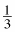that goes through the point (0, 5).

2. The line parallel to y = 2x − 5 that goes through the point (1, 7).

3. The line perpendicular to y = 2x − 5 that goes through the point (1, 7).

4. The line that goes through the point (0, 0) so that the tangent of the angle it makes with the x-axis is 2.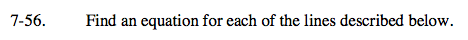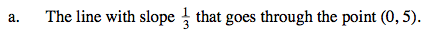Use slope-intercept form.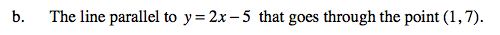Parallel lines have the same slope. Use the point-slope form of the equation of a line.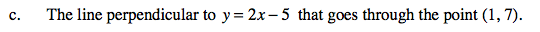The slopes of perpendicular lines are opposite reciprocals.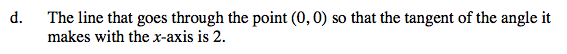Remember the trigonometric relationships.

How might tangent's relationship to the opposite and adjacent sides relate to the rise and run of the slope?